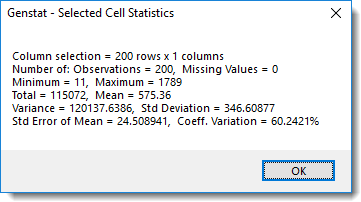1. Home
2. Summarizing Selected Cells

# Summarizing Selected Cells

You can calculate summary statistics for a selection of cells. These include the arrangement of cells (selection types, and number of rows by columns), the number of numerical observations, missing values and text cells, and for the numerical cells, the total, mean, minimum, maximum, variance, standard deviation, standard error of the mean and the % coefficient of variation.

1. Make a cell selection then from the menu select Spread | Calculate | Cell Selection Stats.Updated on December 14, 2018